# Diagrammatic algebra: On the road to category theory

Aryan Ghobadi gives a maths lecture at a zooImage: Highways Agency, CC BY 2.0

As trends go, diagrammatic algebra has taken mathematics by storm. Appearing in papers on computer science, pure mathematics and theoretical physics, the concept has expanded well beyond its birthplace, the theory of Hopf algebras. Some use these diagrams to depict difficult processes in quantum mechanics; others use them to model grammar in the English language! In algebra, such diagrams provide a platform to prove difficult ring theoretic statements by simple pictures.

As an algebraist, I’d like to present you with a down-to-earth introduction to the world of diagrammatic algebra, by diagrammatising a rather simple structure: namely, the set of natural numbers! At the end, I will allude to the connections between these diagrams and the exciting world of higher and monoidal categories.

Now—imagine yourself in a lecture room, with many others as excited about diagrams as you (yes?!), plus a cranky audience member, who isn’t a fan of category theory, in the front row:What we would like to draw today is the process of multiplication for the natural numbers. In its essence, multiplication, $\times$, takes two natural numbers, say 2 and 3, and produces another natural number…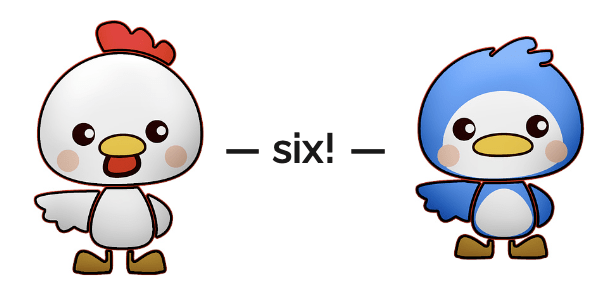Because it takes two elements and produces just one, multiplication is formally called a binary operation: we can say it is a function $m:\mathbb{N}\times\mathbb{N}\to\mathbb{N}$, where, for example, $m(2,3)=6$.

We will keep this $m$ notation for natural number multiplication to avoid confusion with the so-called product of two sets $A$ and $B$, which is the set of all possible pairs from $A$ and $B$ and is denoted by
$A \times B = \{(a,b) : a \in A, \, b \in B\}$

Now we draw (reading diagrams from top to bottom):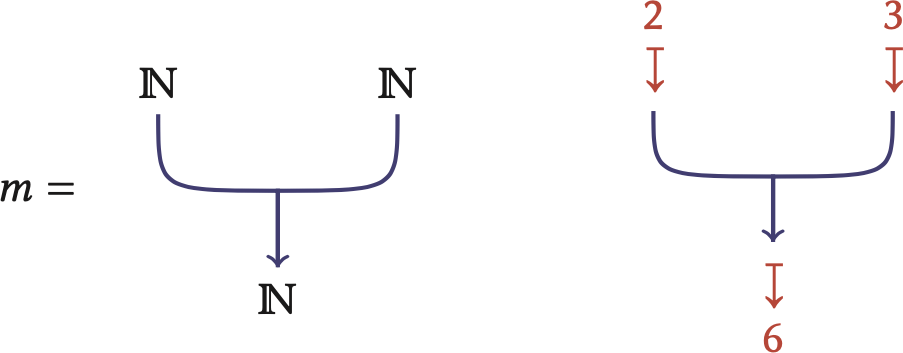Multiplication, $m$, can really be thought of as a ‘meta-road’: it’s a one-way road with two entry lanes, both departing from two cities whose cars correspond to natural numbers, and one exit lane leading to natural-number-land again.
We call our roads ‘meta’ because two cars, 2 and 3, enter the lanes at the same time, possibly colliding in the middle, passing through time and space, and a brand new car, 6, exits into the city.Do not be alarmed by this interruption! I am ready to respond.

## Diagrams for a monoid

A monoid structure is a fancy word for some of the nice properties that the multiplication of natural numbers satisfies:

1. associativity: $m(x,m(y,z)) = m(m(x,y),z),$eg $2 \times (3\times 5) = 30 = (2\times 3) \times 5$
2. a unit element exists: $m(1,x) = x = m(x,1),$eg $1\times x = x = x \times 1 \; \forall x \in \mathbb{N}$

Now we simply visualise these properties using our pictorial notation. Associativity translates to these compound meta-roads being the same:But why are the diagrams the same? The key ingredient is that we need to put on our topological glasses! We don’t care about length or curvature in our roads. It’s as if the asphalt moves freely above the sand! With our new glasses, all the following diagrams are the same and the middle lane can move freely from one side to the other: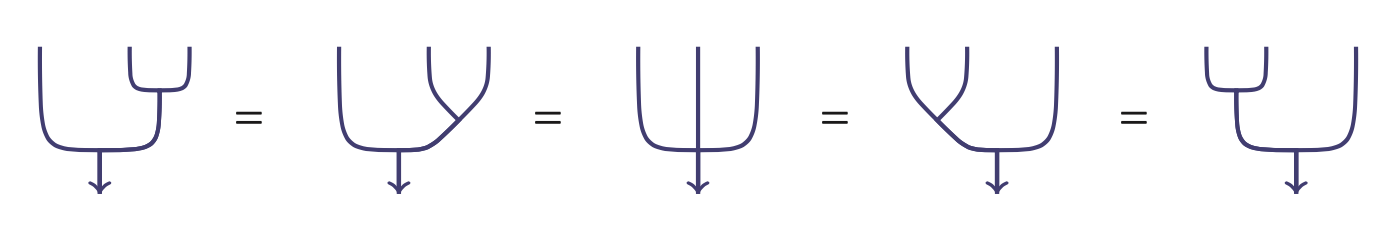The second property we need to visualise is the unit element $1 \in \mathbb{N}$. In previous diagrams, any car from $\mathbb{N}$ can use the roads, whereas to discuss multiplication by 1, we need a unique car to use the road. So we draw a special diagramfor the road where only the car corresponding to 1 can use the lane. The unit conditions require one more ingredient. Each city can have a boring ‘identity road’ $\mathrm{id}$, where nothing happens to cars taking this road. They simply leave and enter the city looking the same. With this in mind, the diagrams representing the unit condition turn into the following picture:This should not be a surprise since it is natural to think of multiplication by 1, $m(1,x)$ for any $x$, as a function from $\mathbb{N}$ to $\mathbb{N}$, which ultimately sends every number to itself. Putting our topological glasses back on,looks as if the diagram for the identity road grew an extra hair, so we can push it back in!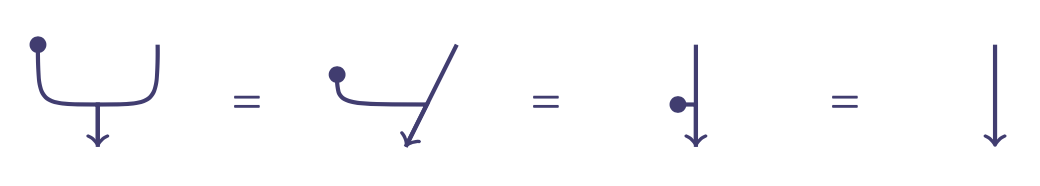In our car metaphor, the left side represents a main road with an additional lane entering it, but this lane is reserved for a ‘harmless’ car that does not interact with any of the other cars. So, it’s the same as if the main road were the identity road, where nothing happens to the cars driving on it.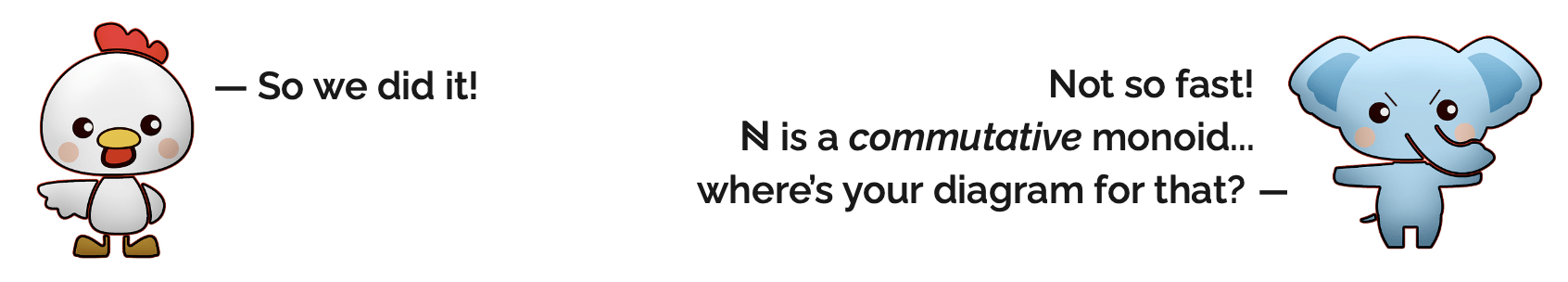Here the cranky listener is using the old trick of deploying fancy words to heckle me. The word commutative just means that the order in which we multiply the numbers doesn’t matter. Formally, $m$ being commutative means
$m(a,b) = m(b,a) \quad \text{for any }a,b\in\mathbb{N}.$
For example, $2 \times 3 = 6 = 3 \times 2$.

To represent this, we need our roads to pass over each other. We need to build bridges! If we can build bridges and allow lanes to pass over each other, ie diagrams like, then commutativity translates to these diagrams being equal: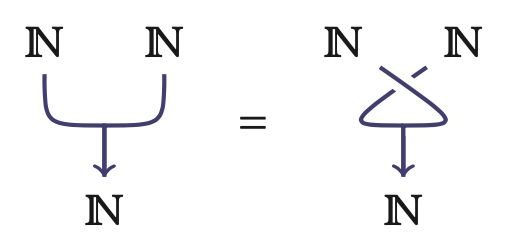To truly see this property, we need to upgrade our glasses to 3D glasses to capture three-dimensional topology. If we view the string diagrams through our 3D glasses, then one could unwind the right-hand diagram by rotating it as so:To placate this restless member of the audience, I will present the punchline a bit early and use the keyword ‘category’ before explaining what it is.

The reason we can draw a commutative monoid such as $\mathbb{N}$ as a three-dimensional diagram is because commutative monoids live in what we call braided categories such as the category of sets. Today’s algebraists will tell you that a braided category is an example of a weirder structure called a 3-category, which has some 3D topology hidden in it. But this takes us into the daunting world of higher categories, and by this point my heckler is hopefully intrigued but has too much pride to ask me to elaborate.Aha! Back to our story…

## Categories

In the same way that looking at the connections between cities in a country is more enlightening than looking at the cities independently, in mathematics it’s more useful to understand the relation between mathematical objects. For example, instead of looking at sets $\mathbb{N}, \mathbb{R}, \{1,2,3\}, \emptyset$, I really need to discuss functions between sets to understand how sets relate to each other. This now fits in a bigger framework, a category. A category has some cities, for example sets $A$, $B$ and $C$, and some roads $f:A \to B$ between the cities, with two extra rules!

1. If roads $f:A \to B$ and $g:B \to C$ are part of my category, then so is a composition road $gf:A \to C$ which is made up from joining roads $f$ and $g$ (first taking the road $f$ to the city $B$ followed by the road $g$):2. Every city should have a special ‘safe’ road, called the identity road, like the identity function $\mathrm{id}_{\mathbb{N}}$ for $\mathbb{N}$: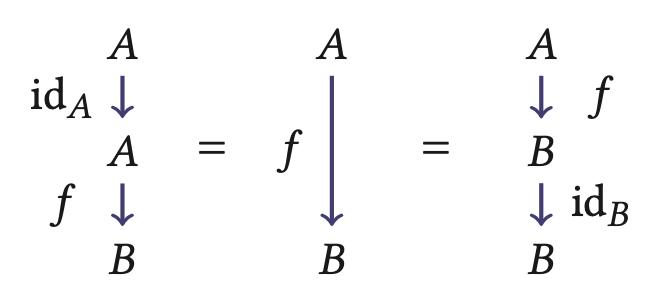Categories provide a platform to draw one-dimensional diagrams and a ‘1D calculus’, ie a way to manipulate these diagrams, as I’ve shown on the right there.

The category of sets has sets as cities and functions as roads. The identity road for each city $A$ is just the identity function $\mathrm{id}_A:A \to A$, where $\mathrm{id}_A(a) = a$ for all $a \in A$.

## Monoidal categories

The missing piece for a 2D calculus is a way to write in the horizontal direction. When we visualised $m:\mathbb{N}\times\mathbb{N}\to\mathbb{N}$ as a diagram, we said that writing two cities $A$ and $B$ next to each other meant the product of the two sets $A \times B$. In other words, writing cities in rows should have a good meaning, where ‘good’ means that roads between these cities can run parallel in the vertical direction. That is, in the case of sets, for every pair of functions $f:A_1 \to A_2$ and $g:B_1 \to B_2$, we have a new function $f \times g:A_1 \times B_1 \to A_2 \times B_2$. In our diagrams, we represent the road $f \times g$ by the roads $f$ and $g$ running parallel: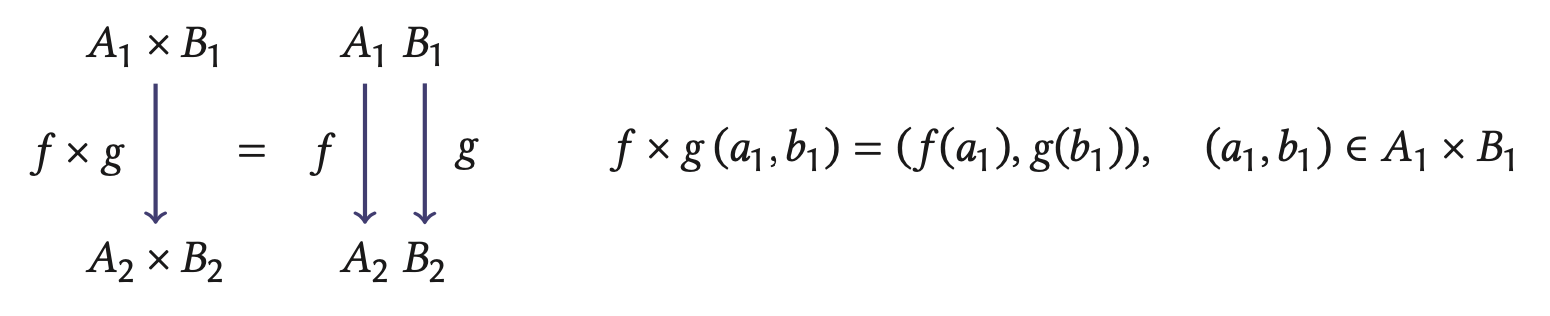Similar to the identity roads acting as ineffective components in the vertical direction, we require an ’empty city’ $E$ which behaves indifferently in the horizontal direction:
$A\;E \; = \; A \; = \; E\;A.$
A bit more formally, for each pair of objects $A$ and $B$, the object ‘$A$ next to $B$’ is written as $A \otimes B$. Parallel roads are written as $f \otimes g$ and $E$ is called the unit. A category with an $\otimes$ operation on pairs of cities and roads and a unit $E$ is called monoidal. It should be clear that monoidal categories provide a setting for 2-dimensional diagrams: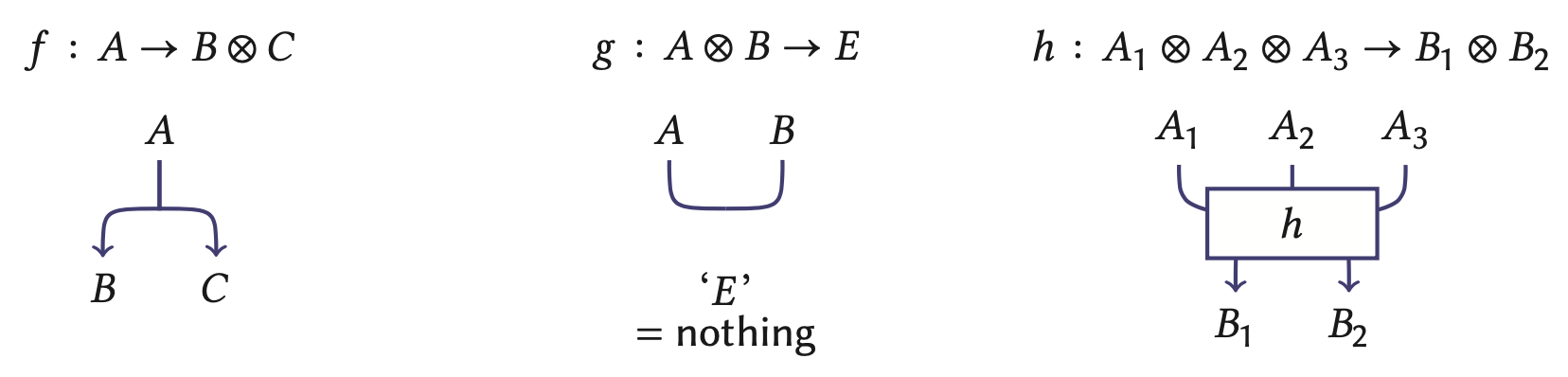The monoidal structure on the category of sets is given by $A \otimes B = A \times B$, $f \otimes g = f \times g$; and $E = \{*\}$ is the set with one element, so that $\{*\} \times A = \{(*,a):a \in A\}$.

By now the room is probably silent and the fear that the audience has long drifted off into sweet dreams of differential equations dawns on me. But…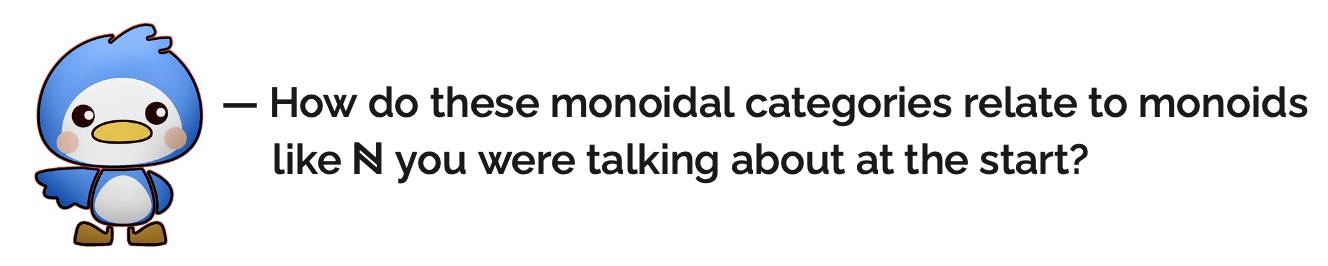An intelligent question!

In the same way you call a set a monoid when you can multiply its elements, a category is called monoidal when you can ‘multiply’ its cities and roads, and instead of a unit element you have a unit city. A trendier way to say this is “monoidal categories categorify monoids”. This is reflected in the fact that a monoid structure on an object of a category only makes sense when the category itself has a monoidal structure.

## Braided monoidal categories

In a braided category, the order of cities in a row can be swapped! To swap any two cities $A$ and $B$, we need a method of travel—a road—from $A \otimes B$ to $B \otimes A$. These roads should have two entry lanes from the cities $A$ and $B$, and two exit lanes into $B$ and $A$, in that order. We’d also like these roads, which we denote by $b_{A,B}$, to resemble the 3D picture, which we saw when describing the commutative property of $\mathbb{N}$. The next rules which need to be satisfied are directly influenced by topology.Firstly, each pass over road $b_{A,B}$ should also be invertible by a road $b_{A,B}^{-1}$ resembling the move. As apparent in the diagram on the right, the composition of two such roads should be the same as the identity roads of $A$ and $B$ running parallel.

The other conditions which need to hold just mean that if you take a number of cities $(A,B,C)$ and reorder them (maybe to $C,B,A$) via such passover roads, the outcome should be the same journey:Geometrically this translates to ‘the order in which the roads lay above each other matters, not the order in which one passes over the other’. As in this picture, the road connected to $A$ lies above the road connected to $B$, which itself lies above the road connected to $C$. However, the order in which they pass over each other does not matter.

A monoidal category with passover roads for any pair of cities, as described above, is called braided. In the category of sets, the passover roads for sets $A$ and $B$ are provided by
$b_{A,B}:A \times B \to B \times A, \quad b_{A,B}(a,b) = (b,a), \quad a\in A, b \in B.$
For those with some university algebra knowledge, another important example of braided monoidal categories is the category of vector spaces with the tensor product of vector spaces. This is in fact where the notation $\otimes$ comes from.Well…

## The big finale… higher algebra!

Let’s say we want to describe a larger system than cities and roads between them. We really want to know how two roads $f,g$ between two cities $A,B$ are related to each other. Under this geographical metaphor, this would entail looking at which streets connect the two roads within the two cities:We call such a pair of streets connecting roads $f$ and $g$ a 2-road between $f$ and $g$. A 2-category carries the information of cities, roads and 2-roads (for those not entertained by my metaphors: objects, morphisms and 2-morphisms) where we draw roads and 2-roads by $\rightarrow$ and $\Rightarrow$, respectively. Similarly to how we can compose ordinary roads, we compose 2-roads $\theta: f \Rightarrow g$ and $\eta:g \Rightarrow h$ ‘vertically’ to produce a new 2-road $\eta\circ_v\theta: f \Rightarrow h$:We can only do this when $f$, $g$ and $h$ are all roads between the same two cities $A,B$.

But in addition to this vertical composition, 2-roads also have a horizontal composition:Such compositions need to act well together, ie the order of composing horizontally or vertically should not matter: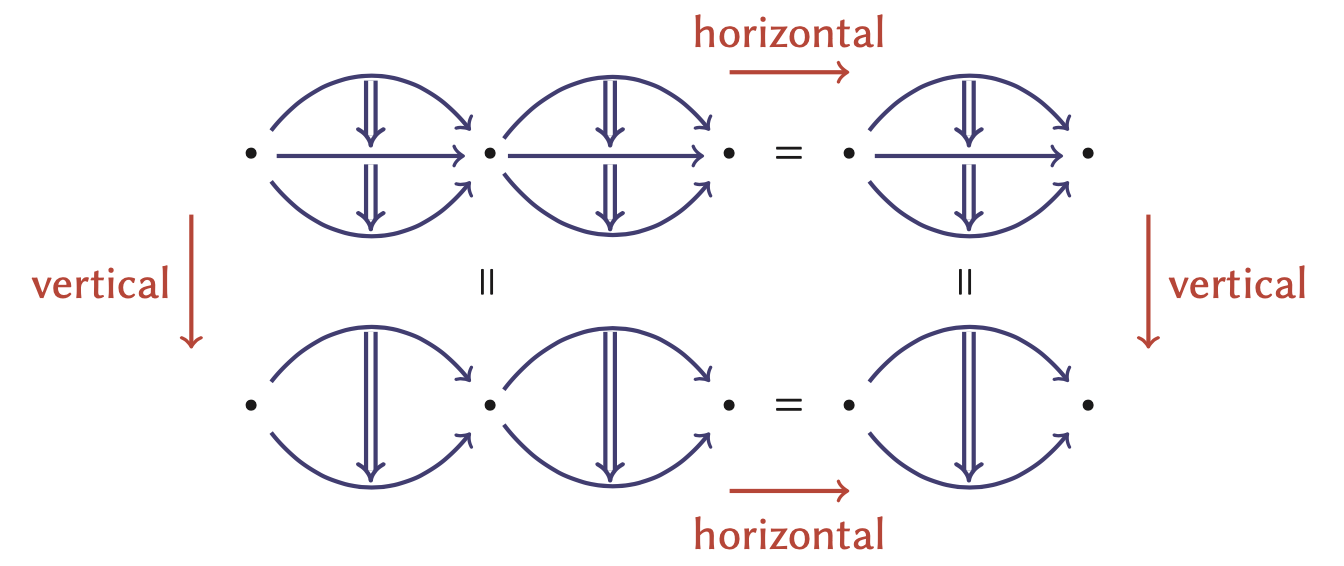Diagrams like the above provide a platform for a 2-dimensional calculus as well and this is no coincidence. The information for a monoidal category is equivalent to the information needed for a 2-category with a single city. To better understand this, compare the pictures we have been drawing:

monoidal category equivalent to
2-category with one city $*$
cities, eg $A$ roads from $*$ to $*$, eg $A$
roads, eg $m$ 2-roads, eg $m$
monoidal operation $\otimes$ for cities roads composing
roads running parallel: $\otimes$ for roads horizontal composition
empty city identity road from $*$ to $*$The diagram on the right shows how information transfers between the two settings. This brings us back to why we can draw a commutative monoid, such as the natural numbers, via 3D diagrams. First remember that to talk about a monoid being commutative, we needed to be able to swap elements. So we really need a braided monoidal category. In a similar fashion to how monoidal categories are 2-categories in disguise, a braided category is a 3-category with one city and one road, and provides a 3D calculus, where our commutative monoid $\mathbb{N}$ can live!So maybe now while these cheers fill the air, my heckler walks out of the lecture room and slams the door. I smile with pride, knowing that ‘category theory won today’.

No mathematicians were harmed during the making of this article. All audience members were fictitious and no real mathematicians were forced to attend my lecture.Aryan is a PhD student in mathematics at Queen Mary University of London, working with categories in quantum algebra. He is often the cranky audience member in the front row.